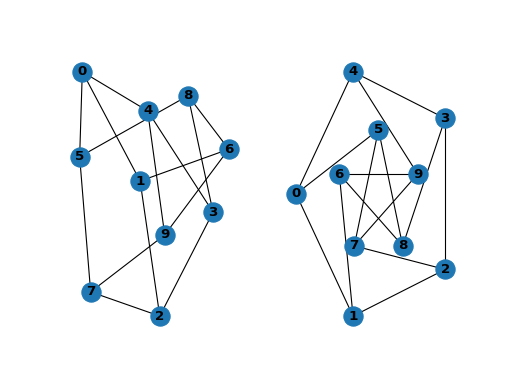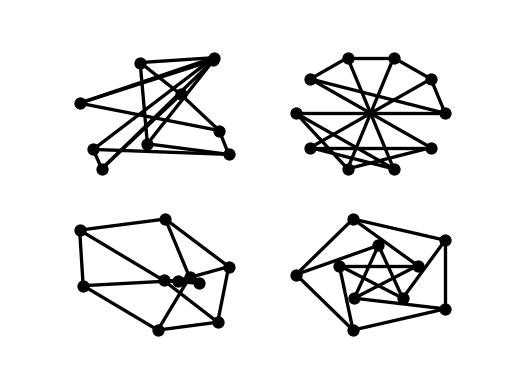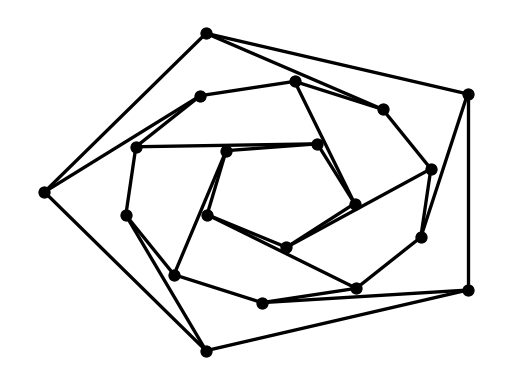# 教程¶

## 创建图形¶

>>> import networkx as nx
>>> G = nx.Graph()


Python 的 None 对象不应用作节点，因为它确定是否在许多函数中分配了可选函数参数。

## 结点¶

G 可以通过多种方式生长。NetworkX包括许多图形生成器功能和以多种格式读取和写入图形的工具。我们先来看看简单的操作。您可以一次添加一个节点，

>>> G.add_node(1)


>>> G.add_nodes_from([2, 3])


>>> H = nx.path_graph(10)


>>> G.add_node(H)


G 现在包含 H 作为节点。这种灵活性非常强大，因为它允许图形、文件图形、函数图形等等。值得考虑如何构造应用程序，使节点成为有用的实体。当然，在 G 如果您愿意的话，还可以使用一个由标识符为节点信息键入的独立字典。

## 边缘¶

G 也可以通过一次添加一个边来生长，

>>> G.add_edge(1, 2)
>>> e = (2, 3)
>>> G.add_edge(*e)  # unpack edge tuple*


>>> G.add_edges_from([(1, 2), (1, 3)])


>>> G.add_edges_from(H.edges)


>>> G.clear()


>>> G.add_edges_from([(1, 2), (1, 3)])


>>> G.number_of_nodes()
8
>>> G.number_of_edges()
3


>>> list(G.nodes)
[1, 2, 3, 'spam', 's', 'p', 'a', 'm']
>>> list(G.edges)
[(1, 2), (1, 3), (3, 'm')]
[2, 3]
>>> G.degree  # the number of edges incident to 1
2


>>> G.edges([2, 'm'])
EdgeDataView([(2, 1), ('m', 3)])
>>> G.degree([2, 3])
DegreeView({2: 1, 3: 2})


>>> G.remove_node(2)
>>> G.remove_nodes_from("spam")
>>> list(G.nodes)
[1, 3, 'spam']
>>> G.remove_edge(1, 3)


>>> G.add_edge(1, 2)
>>> H = nx.DiGraph(G)   # create a DiGraph using the connections from G
>>> list(H.edges())
[(1, 2), (2, 1)]
>>> edgelist = [(0, 1), (1, 2), (2, 3)]
>>> H = nx.Graph(edgelist)


## 访问边缘和邻居¶

>>> G  # same as G.adj
AtlasView({2: {}})
>>> G
{}
>>> G.edges[1, 2]
{}


>>> G.add_edge(1, 3)
>>> G['color'] = "blue"
>>> G.edges[1, 2]['color'] = "red"


>>> FG = nx.Graph()
>>> FG.add_weighted_edges_from([(1, 2, 0.125), (1, 3, 0.75), (2, 4, 1.2), (3, 4, 0.375)])
>>> for n, nbrs in FG.adj.items():
...    for nbr, eattr in nbrs.items():
...        wt = eattr['weight']
...        if wt < 0.5: print('(%d, %d, %.3f)' % (n, nbr, wt))
(1, 2, 0.125)
(2, 1, 0.125)
(3, 4, 0.375)
(4, 3, 0.375)


>>> for (u, v, wt) in FG.edges.data('weight'):
...     if wt < 0.5: print('(%d, %d, %.3f)' % (u, v, wt))
(1, 2, 0.125)
(3, 4, 0.375)


## 向图形、节点和边添加属性¶

### 图形属性¶

>>> G = nx.Graph(day="Friday")
>>> G.graph
{'day': 'Friday'}


>>> G.graph['day'] = "Monday"
>>> G.graph
{'day': 'Monday'}


### 节点属性¶

>>> G.add_node(1, time='5pm')
>>> G.nodes
{'time': '5pm'}
>>> G.nodes['room'] = 714
>>> G.nodes.data()
NodeDataView({1: {'time': '5pm', 'room': 714}, 3: {'time': '2pm'}})


### 边缘属性¶

>>> G.add_edge(1, 2, weight=4.7 )
>>> G.add_edges_from([(3, 4), (4, 5)], color='red')
>>> G.add_edges_from([(1, 2, {'color': 'blue'}), (2, 3, {'weight': 8})])
>>> G['weight'] = 4.7
>>> G.edges[3, 4]['weight'] = 4.2


## 有向图¶

>>> DG = nx.DiGraph()
>>> DG.add_weighted_edges_from([(1, 2, 0.5), (3, 1, 0.75)])
>>> DG.out_degree(1, weight='weight')
0.5
>>> DG.degree(1, weight='weight')
1.25
>>> list(DG.successors(1))

>>> list(DG.neighbors(1))



>>> H = nx.Graph(G)  # convert G to undirected graph


## 多重图¶

NetworkX为允许任意节点对之间存在多个边的图形提供类。这个 MultiGraphMultiDiGraph 类允许您两次添加相同的边缘，可能使用不同的边缘数据。这对某些应用程序来说可能很强大，但许多算法在此类图上没有很好的定义。如果结果定义明确，例如： MultiGraph.degree() 我们提供功能。否则，您应该以一种使测量定义良好的方式转换为标准图。

>>> MG = nx.MultiGraph()
>>> MG.add_weighted_edges_from([(1, 2, 0.5), (1, 2, 0.75), (2, 3, 0.5)])
>>> dict(MG.degree(weight='weight'))
{1: 1.25, 2: 1.75, 3: 0.5}
>>> GG = nx.Graph()
>>> for n, nbrs in MG.adjacency():
...    for nbr, edict in nbrs.items():
...        minvalue = min([d['weight'] for d in edict.values()])
...        GG.add_edge(n, nbr, weight = minvalue)
...
>>> nx.shortest_path(GG, 1, 3)
[1, 2, 3]


## 图形生成器和图形操作¶

1. 应用经典图形操作，例如：

subgraph(G, nbunch)      - induced subgraph view of G on nodes in nbunch
union(G1,G2)             - graph union
disjoint_union(G1,G2)    - graph union assuming all nodes are different
cartesian_product(G1,G2) - return Cartesian product graph
compose(G1,G2)           - combine graphs identifying nodes common to both
complement(G)            - graph complement
create_empty_copy(G)     - return an empty copy of the same graph class
to_undirected(G) - return an undirected representation of G
to_directed(G)   - return a directed representation of G

2. 调用一个经典的小图，例如，

>>> petersen = nx.petersen_graph()
>>> tutte = nx.tutte_graph()
>>> maze = nx.sedgewick_maze_graph()
>>> tet = nx.tetrahedral_graph()

1. 对经典图形使用（构造）生成器，例如，

>>> K_5 = nx.complete_graph(5)
>>> K_3_5 = nx.complete_bipartite_graph(3, 5)
>>> barbell = nx.barbell_graph(10, 10)
>>> lollipop = nx.lollipop_graph(10, 20)

1. 使用随机图生成器，例如，

>>> er = nx.erdos_renyi_graph(100, 0.15)
>>> ws = nx.watts_strogatz_graph(30, 3, 0.1)
>>> ba = nx.barabasi_albert_graph(100, 5)
>>> red = nx.random_lobster(100, 0.9, 0.9)

1. 使用常用的图形格式读取存储在文件中的图形，如边列表、邻接列表、gml、graphml、pickle、leda等。

>>> nx.write_gml(red, "path.to.file")


## 分析图形¶

>>> G = nx.Graph()
>>> list(nx.connected_components(G))
[{1, 2, 3}, {'spam'}]
>>> sorted(d for n, d in G.degree())
[0, 1, 1, 2]
>>> nx.clustering(G)
{1: 0, 2: 0, 3: 0, 'spam': 0}


>>> sp = dict(nx.all_pairs_shortest_path(G))
>>> sp
{3: , 1: [3, 1], 2: [3, 1, 2]}


## 图形绘制¶

networkx主要不是一个图形绘制包，而是一个带有matplotlib的基本绘图，以及一个使用开源graphviz软件包的接口。这些是 networkx.drawing 模块，如果可能，将导入。

>>> import matplotlib.pyplot as plt


>>> G = nx.petersen_graph()
>>> plt.subplot(121)
<matplotlib.axes._subplots.AxesSubplot object at ...>
>>> nx.draw(G, with_labels=True, font_weight='bold')
>>> plt.subplot(122)
<matplotlib.axes._subplots.AxesSubplot object at ...>
>>> nx.draw_shell(G, nlist=[range(5, 10), range(5)], with_labels=True, font_weight='bold')


(png, hires.png, pdf)>>> plt.show()


>>> options = {
...     'node_color': 'black',
...     'node_size': 100,
...     'width': 3,
... }
>>> plt.subplot(221)
<matplotlib.axes._subplots.AxesSubplot object at ...>
>>> nx.draw_random(G, **options)
>>> plt.subplot(222)
<matplotlib.axes._subplots.AxesSubplot object at ...>
>>> nx.draw_circular(G, **options)
>>> plt.subplot(223)
<matplotlib.axes._subplots.AxesSubplot object at ...>
>>> nx.draw_spectral(G, **options)
>>> plt.subplot(224)
<matplotlib.axes._subplots.AxesSubplot object at ...>
>>> nx.draw_shell(G, nlist=[range(5,10), range(5)], **options)


(png, hires.png, pdf)>>> G = nx.dodecahedral_graph()
>>> shells = [[2, 3, 4, 5, 6], [8, 1, 0, 19, 18, 17, 16, 15, 14, 7], [9, 10, 11, 12, 13]]
>>> nx.draw_shell(G, nlist=shells, **options)


(png, hires.png, pdf)>>> nx.draw(G)
>>> plt.savefig("path.png")


>>> from networkx.drawing.nx_pydot import write_dot
>>> pos = nx.nx_agraph.graphviz_layout(G)
>>> nx.draw(G, pos=pos)
>>> write_dot(G, 'file.dot')